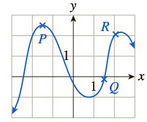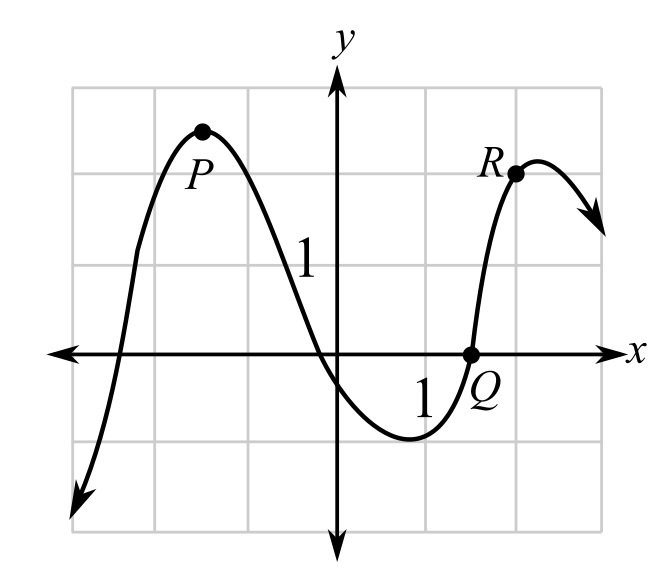Chapter 10.5, Problem 26EFinite Mathematics and Applied Cal...

7th Edition
Stefan Waner + 1 other
ISBN: 9781337274203

Solutions

Chapter
SectionFinite Mathematics and Applied Cal...

7th Edition
Stefan Waner + 1 other
ISBN: 9781337274203
Textbook Problem

In each of Exercises 23–26, three slopes are given. For each slope, determine at which of the labeled points on the graph the tangent line has that slope.a. 0 b. 3 c. 1(a)

To determine

The labelled points on the graph that has a value of the slope of a tangent as 0.Explanation

Given information:

The provided graph:

When a function is increasing or rising the slope of the function is positive and greatest where the tangent is more slanted whereas when the function is decreasing or falling its slope is negative and it has the least value where the tangent is the most slanted.

From the graph, one can observe that the function is first increasing then the function takes a rebound and start...

(b)

To determine

The labelled points on the graph that has a value of the slope of a tangent as 3.(c)

To determine

The labelled points on the graph that has a value of the slope of a tangent as 1.

The provided graph:Still sussing out bartleby?

Check out a sample textbook solution.

See a sample solution

The Solution to Your Study Problems

Bartleby provides explanations to thousands of textbook problems written by our experts, many with advanced degrees!

Get Started

Evaluate limnn2i=1nj=1n21n2+ni+j.

Calculus (MindTap Course List)

20. If a production function is given by , find the marginal productivity of (a) x. (b) y.

Mathematical Applications for the Management, Life, and Social Sciences

Find the general indefinite integral. (sinx+sinhx)dx

Single Variable Calculus: Early Transcendentals

The slope of the tangent line to r = cos θ at is:

Study Guide for Stewart's Multivariable Calculus, 8th